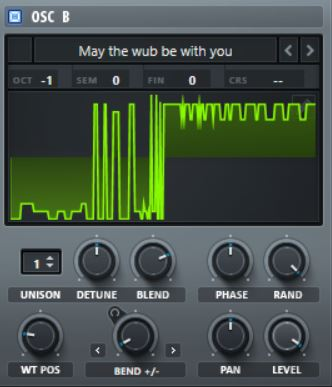# Converting a wav file into a wavetable, no waveform in plot?

Hello againI’ve created a single cycle waveform as a .wav file and I’m trying to convert it into a wavetable
to be loaded into an oscillator in a synthdef.

So far I’ve been able to get the following code to run but when I plot the final buffer the wavetable is loaded into I get a graph with nothing in it.

The float array values all seem to be 0 as well, so it’s not actually turning the amplitude information from the .wav file into values in the array. Its created an array of 2056 values, which seem like the right number for the waveform since it’s literally one cycle (maybe a tiny fraction of a second long at 44.1 sample rate).

Why would the values all be 0?

The code is

``````b = SoundFile.openRead("C:\\Users\\44774\\AppData\\Local\\SuperCollider\\Tables\\wubsquare.wav".standardizePath);
a = FloatArray.newClear(b.numFrames);
c = a.as(Signal);
d = c.asWavetable;

``````

You can see I’ve opened the file, then sent it to an array, then converted the array into a signal, then converted the signal into a wavteable, and finally loaded that wavetable onto a buffer.

I tried running
d.plot;

and
e.plot;

And get just a flat graph which is basically silence, if I’m not mistaken?

Does anyone have any ideas on why that is?
Maybe there’s a better way to achieve what I’m trying to do?

Any help or suggestions are greatly appreciated!
Thanks!

Not exactly. You’ve created an array that’s the same size as the file, but you haven’t read the file’s contents. See the help file for the readData method.

Edit: Oh, I see what the confusion may have been. `openRead` means open for reading (as opposed to `openWrite`) but doesn’t mean open-and-read-contents. It prepares for reading contents but doesn’t actually read them.

hjh

Dude! Thank you!
I got it to work!

You were right, I needed to use .readData AFTER using .openRead and creating the array.

I plotted the final wavetable and it matches the original wavetable I created in Serum.

Here’s the working code:

``````indent preformatted text by 4 spaces
// variable 'a' to open the sound file to prepare for reading contents
// variable 'b' to create a float array that is the same size as the sound file
b = FloatArray.newClear(a.numFrames);
// variable 'c' will read the sample data of the sound file 'a' into the array provided as variable 'b'
// variable 'd' will convert the array set as variable 'b' (which should now contain the data from the sample file) into a signal
d = b.as(Signal);
// variable 'e' will convert the signal produced from the step in variable 'd' into a wavetable format
e = d.asWavetable;
// variable 'f' will load the wavetable created in the previous step in variable 'e' into a buffer on the server

e.plot;
``````

Now I just need to send the wavetable in the buffer to an Osc in a synthdef.
Yay, baby steps!

Here’s the plotted wavetable:

And here’s the original wavetable created in SerumThanks again for your help and suggestions!